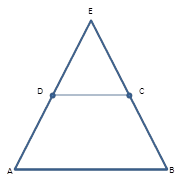Chapter 4.4, Problem 18EElementary Geometry For College St...

7th Edition
Alexander + 2 others
ISBN: 9781337614085

Solutions

Chapter
SectionElementary Geometry For College St...

7th Edition
Alexander + 2 others
ISBN: 9781337614085
Textbook Problem

Given: Isosceles Δ A B E with A E ¯ ≅ B E ¯ ; also, D and C are midpoints of A E ¯ and B E ¯ , respectively.Prove: ABCD is an isosceles trapezoid.Exercises 17, 18

To determine

To Prove:

The trapezoid is isosceles.

Explanation

Consider the following diagram.

Given: Isosceles ΔABE with AE¯BE¯, also D and C are midpoints of AE¯ and BE¯, respectively.

Prove: ABCD is an isosceles trapezoid

Still sussing out bartleby?

Check out a sample textbook solution.

See a sample solution

The Solution to Your Study Problems

Bartleby provides explanations to thousands of textbook problems written by our experts, many with advanced degrees!

Get Started

Use the guidelines of Section 4.5 to sketch the curve. y=x1x2

Single Variable Calculus: Early Transcendentals, Volume I

Let h be the function defined by h(x) = x3 x2 + x + 1. Find h(5), h(0), h(a), and h(a).

Applied Calculus for the Managerial, Life, and Social Sciences: A Brief Approach

is the binomial series for:

Study Guide for Stewart's Single Variable Calculus: Early Transcendentals, 8th